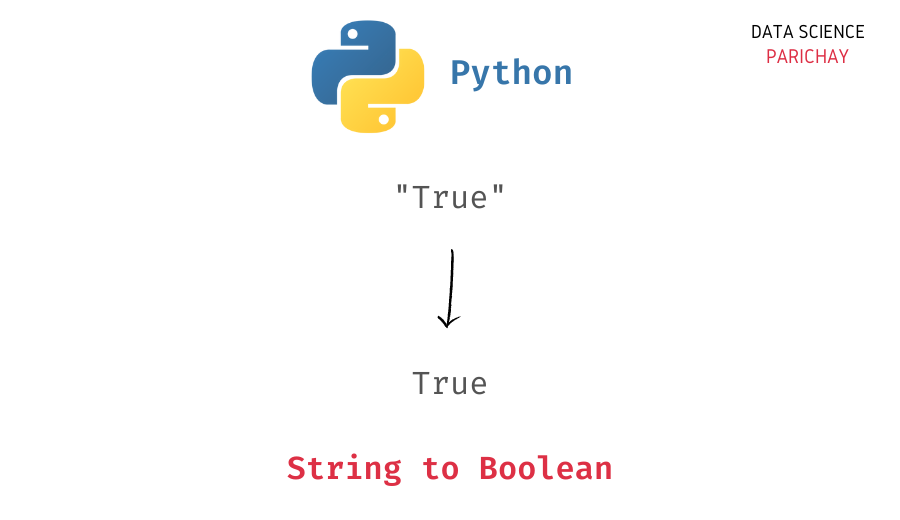# Python – Convert String to Boolean

In this tutorial, we will look at how to convert a string to a boolean in Python with the help of some examples.

## How to convert string to a boolean in Python?

You can use the built-in `bool()` function to convert a string to a boolean in Python. Pass the string as an argument to the function. The following is the syntax –

```# string to boolean
bool(s)```

It returns `True` for non-empty string and `False` for empty strings.

Let’s look at some examples of using the above function.

```# create a string
s = "cat"
# convert to boolean
print(bool(s))```

Output:

`True`

We get `True` as the output because the string `s` above is non-empty.

Let’s look at another example, this time with an empty string.

```# create a string
s = ""
# convert to boolean
print(bool(s))```

Output:

📚 Data Science Programs By Skill Level

Introductory

Intermediate ⭐⭐⭐

🔎 Find Data Science Programs 👨‍💻 111,889 already enrolled

Disclaimer: Data Science Parichay is reader supported. When you purchase a course through a link on this site, we may earn a small commission at no additional cost to you. Earned commissions help support this website and its team of writers.

`False`

We get `False` as the output.

Note that the result from the `bool()` function depends on whether the passed string is empty or not. So even if you pass truth values in string form, for example, “True” or “False”, you’ll get the same result.

```# create string
s1 = "True"
s2 = "False"
# convert to boolean
print(bool(s1))
print(bool(s2))```

Output:

```True
True```

We get `True` as the output for both the strings.

## Convert string truth values to boolean in Python

If you want to convert a string containing truth values such as “True” or “False” to boolean, you can use the equality operator to compare them with “True” and “False” and return a boolean value.

Let’s look at an example.

```# string to boolean function
def str_to_bool(s):
if s == "True":
return True
elif s == "False":
return False
else:
return None

# create string
s1 = "True"
s2 = "False"
# string to boolean
print(str_to_bool(s1))
print(str_to_bool(s2))```

Output:

```True
False```

We get `True` for the string having the value “True” and `False` for the string having the value “False”.

Alternatively, if you want a more exhaustive match for a string containing truth values like “True”, “1”, “TRUE”, “true”, etc. you can use the membership operator `in`. Let’s look at an example.

```# string to boolean function
def str_to_bool(s):
if s in ("True", "TRUE", "true", "1"):
return True
elif s in ("False", "FALSE", "false", "0"):
return False
else:
return None

# list of strings
s_ls = ["True", "TRUE", "1", "False", "FALSE", "0"]
# string to boolean
for s in s_ls:
print(str_to_bool(s))```

Output:

```True
True
True
False
False
False```

We get `True` as the output for the string “true”.

Note that the above example is a very specific use case. It all depends on which string values you want to consider as `True` and which string values you want to consider as `False`.

You might also be interested in –

•